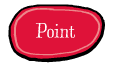# RPoint¶## Usage¶

```# robofab manual
# Point object
# usage examples

contour = CurrentGlyph()
print contour.points

from random import randint
for p in contour.points:
p.x += randint(-10,10)
p.y += randint(-10,10)

contour.update()
```
```<RPoint for AFont.A>
```

## Description¶

`RPoint` is perhaps the smallest object in RoboFab objects. It represents one single point with a particular coordinate in a contour. It is used to access off-curve and on-curve points alike. It’s cousin, bPoint also provides access to incoming and outgoing bcps. `RPoint` is exclusively only one single point.

## Understanding Contours and outlines¶

The way outline data is organised in RoboFab, and how the various objects relate is described here: understanding contours.

## Attributes¶

`x`

The `x` coordinate of this point.

`y`

The `y` coordinate of this point.

`type`

The `type` of this point.

`selected`

Boolean for selection state, i.e. `True` or `False`.

## UFO only attributes¶

`name`

The name of the point (UFO only).

## Methods¶

`copy`()

Return a deepcopy of the object.

`move`((x, y))

Move the anchor of the `bPoint` to `(x,y)`. The relative coordinates of the `bcpIn` and `bcpOut` will remain the same, which means that in fact, they move the same distance.

`round`()

Round the coordinates to whole integers.

`select`(state=True)

Select this point.

`transform`(matrix)

Transform this point. Use a Transform matrix object to mess with the point.